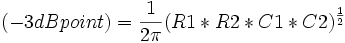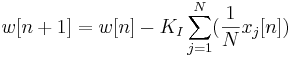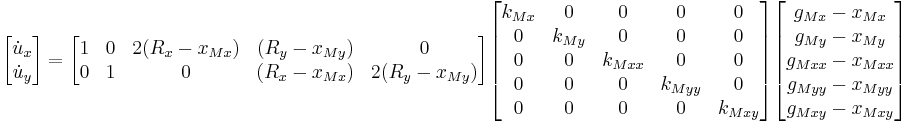The sandbox

(Difference between revisions)

This is the sandbox, so I'm assuming I can edit this as I please.

Test0

Test1

test$(-3dB point) = \frac{1}{2\pi}(R1*R2*C1*C2)^\frac{1}{2}$$x[n+1] = x[n] + \gamma(u[n]-x[n]) - K_P\sum_{j=1}^N(\frac{1}{N}x_j[n]) + K_I\sum_{j=1}^N(\frac{1}{N}w_j[n])$$w[n+1] = w[n] - K_I\sum_{j=1}^N(\frac{1}{N}x_j[n])$$\begin{bmatrix} \dot u_x \\ \dot u_y \\ \end{bmatrix} = \begin{bmatrix} 1 & 0 & 2(R_x-x_{Mx}) & (R_y-x_{My}) & 0 \\ 0 & 1 & 0 & (R_x-x_{Mx}) & 2(R_y-x_{My})\\ \end{bmatrix} \begin{bmatrix} k_{Mx} & 0 & 0 & 0 & 0 \\ 0 & k_{My} & 0 & 0 & 0 \\ 0 & 0 & k_{Mxx} & 0 & 0 \\ 0 & 0 & 0 & k_{Myy} & 0 \\ 0 & 0 & 0 & 0 & k_{Mxy} \\ \end{bmatrix} \begin{bmatrix} g_{Mx}-x_{Mx} \\ g_{My}-x_{My}\\ g_{Mxx}-x_{Mxx} \\ g_{Myy}-x_{Myy} \\ g_{Mxy}-x_{Mxy} \\ \end{bmatrix}$

Testing

Class 1 (W 9/20)

Welcome to the course and course website. Structure of the course (HW due Wed, student-generated lectures and learning materials, in-class assignments, feedback on student lectures, occasional Friday quizzes). Book, software, (lack of) D-H parameters, syllabus, V-REP simulator, office hours.

At home:

Videos: first 3 videos of Chapter 2, through Chapter 2.2
Software: download github software with book, install V-REP and verify that you can use Scenes 1 and 2 (the UR5)
HW1, due 1:30 PM 9/27: Exercises 2.3, 2.9, 2.20, 2.29. Also, create your own example system with closed loops, something not in the book, and solve for the degrees of freedom using Grubler's formula. Make it something that exists or occurs in common experience, not necessarily a robot. Imagine using it to teach someone about Grubler's formula.

Class 2 (F 9/22)

Sample student lecture

At home:

Videos: 2 videos on Chapter 2.3

Class 3 (M 9/25)

Bring your laptop, demo V-REP UR5 scenes
Help with HW1

At home:

Videos: 2 videos, Chapter 2.4 and 2.5
Turn in HW1

Class 4 (W 9/27)

Solutions to HW1; student examples of Grubler's formula

At home:

Videos: first 3 videos of Chapter 3, through Chapter 3.2.1
HW2, due 1:30 PM 10/4:
1) Exercise 3.1, except the y_a axis points in the direction (1,0,0).
2) Exercise 3.2, except p = (1,2,3).
3) Exercise 3.5.
4) Exercise 3.9.
5) In Figure 1.1(a) of the book is an image of a UR5 robot, with a frame at its base and a frame at its end-effector. Eyeballing the end-effector frame, approximately write the rotation matrix that represents the end-effector frame orientation relative to the base frame. Your rotation matrix should satisfy the properties of a rotation matrix (R^T R = I, det(R) = 1). The x-axes are in red, the y-axes are in green, and the z-axes are in blue.
6) Write a program that takes a set of exponential coordinates for rotation from the user as input. It then prints out the following: (a) the corresponding unit rotation axis and the angle of rotation about that axis; (b) the so(3) 3x3 matrix representation of the exponential coordinates; (c) the 3x3 SO(3) rotation matrix corresponding to the exponential coordinates; (d) the inverse of the rotation matrix from (c); (e) the 3x3 so(3) matrix log of the matrix from (d); and (f) the corresponding exponential coordinates for the so(3) matrix (e). Use the code from the book and write your program in Mathematica, MATLAB, or Python. Turn in your code and the output of an example run using (0.5, 1, 0) as the input to part (a).
7) Write a function that returns "true" if a given 3x3 matrix is with a distance epsilon of being a rotation matrix and "false" otherwise. It is up to you to define the "distance" between a random 3x3 real matrix and members of SO(3). Test the function on two matrices, neither of which is exactly in SO(3), but one of which is close (so the result is "true") and one of which is not. Provide the test run output, which also outputs the distance to SO(3) that you defined.
8) Following up on the previous exercise: describe (don't implement, unless you want to) a function that takes a "close by" 3x3 matrix and returns the closest rotation matrix. How would you use the fact that R^T R - I must be equal to zero to modify the initial 3x3 matrix to make it a "close by" rotation matrix? Would the function be iterative?

Class 5 (F 9/29)